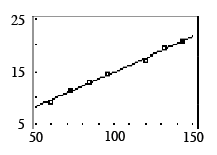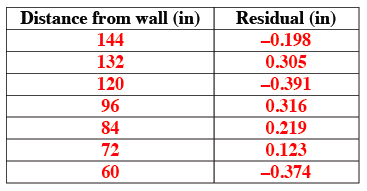### Home > CCA > Chapter 6 > Lesson 6.1.4 > Problem6-42

6-42.

Robbie’s class collected the following view tube data in problem 6-1. Homework Help ✎

 Distancia desde la pared (plg) Amplitud del campo visual (plg) $144$ $20.7$ $132$ 19.6 $120$ 17.3 $96$ 14.8 $84$ 13.1 $72$ 11.4 $60$ 9.3 checksum $708$ checksum 106.2
1. Use your calculator to make a scatterplot and graph the least squares regression line (LSRL). Sketch the graph and LSRL on your paper. Remember to put a scale on the x-axis and y-axis of your sketch. Write the equation of the LSRL rounded to four decimal places.

See scatterplot below. y = 1.6568 + 0.1336x2. With your calculator, find the residuals as you did in part (c) of problem 6‑33. Make a table with the distance from wall (inches) as the first column, and residual (inches) in the second column. What is the sum of the squares of the residuals?

• See table below.
Use your calculator to find the sum of the squares of the residuals.